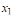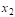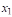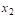### Specifying the data source

Whether you use the Lookup Table wizard or set up the map block manually, you must create the data source, typically referred to as a map file. A map file is a multi-column ASCII data file from which the input signals are mapped to the desired output domain. Signals can be separated by commas, spaces, tabs, vertical bars, colons, semicolons, or slashes.

You can use floating-point or fixed-point values as input. If you index in with fixed-point values, Embed automatically converts to fixed point with optimal scaling. You always enter the table data in floating point.

A map file can be a 1D, 2D, or 3D file.

1D map file

A 1D map file has one independent variable, and can have from one to 16 dependent variable outputs. The first column is an independent variable range. The numbers in the independent variable column must be either increasing in order or decreasing in order, but not both. Each additional data column you supply in the map file yields an additional dependent variable. Use the Edit > Add Connector command to add an output connector for each dependent variable column in the resulting map block.

A one-dimensional matrix is limited to 8,000 rows. Lines that are prefaced with a semi-colon (;) are treated as comments.

2D map file

A 2D map file has two independent variables and one dependent variable output. The first row contains the domain points for the first independent variable; and the first column (excluding the column member in row 1) represents the second independent variable. The position (1,1) must be left blank.

Like one-dimensional mapping, the independent variable values must be either monotically increasing or decreasing.

A two-dimensional matrix is limited to 90 rows by 90 columns (or, a maximum of 89 * 89 data points).

Lines that are prefaced with a semi-colon (;) are treated as comments.

An example of a two-dimensional map file is shown below.

 10 11 20 25 -5 -5 -2 1 20 2 2 5 7 10 3 3 7 8 5 4 4 9 10 2 5 5 11 15 -5

In the above matrix, the first row represents the domain points of the first independent variable, and the first column represents the domain points of the second independent variable. The entries represent the dependent variable values at the corresponding values of independent variables 1 and 2. For example, for= 10,= 2, the output is 2; for= 10.5,= 2.5, the output is 4.25.

3D map file

A 3D map file has three independent variables and one dependent variable output. The format of the first seven lines is as follows:

 Line Format 1 Starts with #3D 2 Indicates the size of dimension 1 3 Indicates the interpolation points of dimension 1 4 Indicates the size of dimension 2 5 Indicates the interpolation of dimension 2 6 Indicates the size of dimension 3 7 Indicates the interpolation of dimension 3

Lines 8 through n are elements of dimension 3 matrices of (dimension 1 columns) * (dimension 2 rows). Lines that are prefaced with double hyphens (--), double slashes (//), or commas (,) are treated as comments.

Dependent variables are linearly interpolated for independent variable values between map points, and linearly extrapolated for values beyond the bounds of the table using the last two points in the table. This feature can be used for static function approximation with measured data or for device calibration, such as thermocouple-voltage-to-temperature conversion.